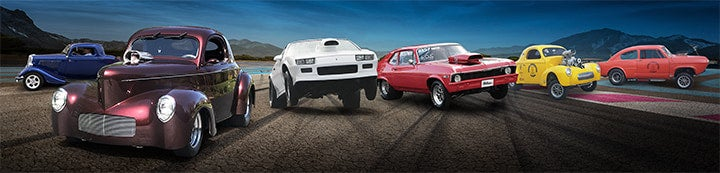1 - 8 of 8 Posts

#### 47chevrolet

·
##### Registered
Joined
·
48 Posts
Discussion Starter
350 .40 over how many cubic inches?

#### BossChevy62

·
##### Registered
Joined
·
21 Posts
350 bored .040 over

a 350 bored .040 over is a 356.88, or a 357 if you wanna round it to the whole number.

#### farna

·
##### Member - AMC/Rambler "guru"
Joined
·
1,783 Posts
Here's the formula:

1/2 of bore (in decimal inches) squared (times itself) x pi x stroke (in inches) x number of cylinders. 3.14 is usually accurate enough for pi.

For a stock Chevy 350 (4.0" bore, 3.48" stroke)

2" x 2" x 3.14 x 3.48" x 8 = 349.67 (rounded to nearest whole number is 350)

Add 0.040 to the bore (4.04) and figure it out. "40 thousandths" is 0.040, NOT 0.40 (that would be "40 hundreths" or "4 tenths" -- nearly half an inch!!). I'm sure that was a typo though.

I could have just done the math for you, but then you'd have to ask again if you decided to bore 0.030" over instead. Now you can just plug the numbers in a calculator (you have one on your computer if nothing else) and go. Remember to add the total amount of overbore then split the bore. You can of course use pi on the scientific view of the computer calculator and come closer by 0.01 or so. But two decimal places is generally enough.

One last thing -- this works for liters as well. Well, actually CC's. Just use the bore and stroke in centimeters. If they are given in millimeters move the decimal place over to the left one zero (100 mm = 10.0 cm). The answer will then be in CC's. 1000 cc is one liter, so move the decimal to the left three places and you have liters (1100 cc = 1.1L).

Okay, enough for math class! can you tell I used to be an instructor??#### toecutter

·
Joined
·
58 Posts
yup!! Can tell. thats some calculating farna :thumbup:

you lost me after, here's the formula#### Blazin72

·
##### You got a leaky spark tube...
Joined
·
2,854 Posts
Another way to figure displacement is Bore x Bore x Stroke x # of cylinders X .7854

It does the same thing that farna showed you but for me it's a little easier to remember.

#### xntrik

·
##### Save a horse, Ride a Cowboy.
Joined
·
5,122 Posts
Blazin72 said:
Another way to figure displacement is Bore x Bore x Stroke x # of cylinders X .7854

It does the same thing that farna showed you but for me it's a little easier to remember.
and easier to do on a cheap calculator since you don't have to remember and re-enter any numbers.

#### rossco

·
##### Registered
Joined
·
30 Posts
Blazin72 said:
Another way to figure displacement is Bore x Bore x Stroke x # of cylinders X .7854

If you then take that answer (which is c.i.d) and divide it by 6, you will get cc's or liters. For example a 350 c.i. divided by 6 is 5833333 or 5800 cc's or 5.8 liter.......A 400 c.i divided by 6 is 6666666 or 6600 cc's or 6.6 liter. About as close as it will take you without being perfect. 350 chevy's are usually regarded as being 5.7 liter.

#### techinspector1

·
##### Registered
Joined
·
17,611 Posts
rossco said:
Blazin72 said:
Another way to figure displacement is Bore x Bore x Stroke x # of cylinders X .7854

If you then take that answer (which is c.i.d) and divide it by 6, you will get cc's or liters. For example a 350 c.i. divided by 6 is 5833333 or 5800 cc's or 5.8 liter.......A 400 c.i divided by 6 is 6666666 or 6600 cc's or 6.6 liter. About as close as it will take you without being perfect. 350 chevy's are usually regarded as being 5.7 liter.
This works better for finding liters from inches if you divide inches by 61. Ex: 350 divided by 61 = 5.73
To find cc's exactly, multiply inches by 16.387 Ex:350 times 16.387 = 5735.
Normally, to find the static compression ratio of a motor, we will use cc's to calculate it. We would therefore use the volume of a cylinder such as a standard bore 350 as follows: .7854 times 4 times 4 times 3.48 times 16.387= 716 cc's in the cylinder.

1 - 8 of 8 Posts